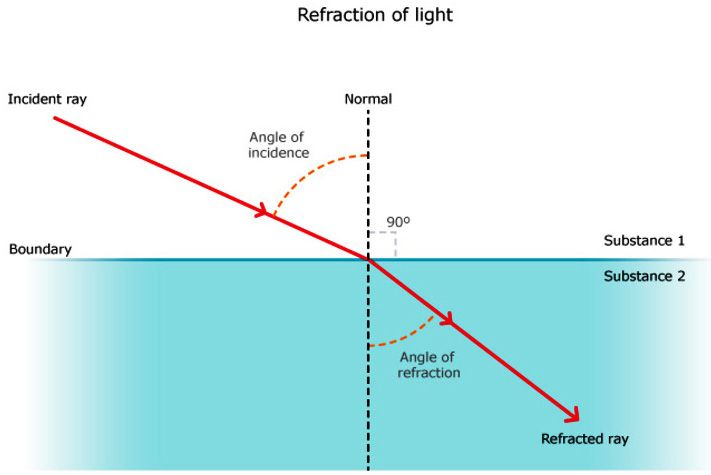We know that light is a form of energy and can undergo various phenomena like diffraction, reflection, refraction, interference, and polarization. Refraction is the phenomenon that takes place due to the bending of light when it travels from medium to another. In this article, let us briefly understand the process of refraction.

Refraction is the bending of a wave when it passes from one medium to another. The bending is caused due to the differences in density between the two substances.

## Defining Refraction

“Refraction is the change in the direction of a wave passing from one medium to another.”

This is one of the most commonly observed phenomena, but other waves like sound waves and water waves also experience refraction. Refraction makes it possible for us to have optical instruments such as magnifying glasses, lenses and prisms. It is also because of the refraction of light that we are able to focus light on our retina.

Refraction in Nature

Why do stars twinkle?

Did you know that the twinkling effect of stars is due to atmospheric refraction? The starlight undergoes several refractions while reaching the Earth. This atmospheric refraction occurs in a medium of gradually changing refractive index. If you are wondering what are the causes of refraction? Pritish Kumar Halder explains the causes of refraction below:

## Causes of Refraction

Change of Speed Results in Change in Direction

A light ray refracts whenever it travels at an angle into a medium of different refractive index. This change in speed results in a change in direction. As an example, consider air travelling into water. The speed of light decreases as it continues to travel at a different angle.

When light travels from air into glass, the light slows down and changes direction slightly. When light travels from a less dense substance to a denser substance, the refracted light bends more towards the normal line. If the light wave approaches the boundary in a perpendicular direction, the light ray doesn’t refract despite the change in speed.

## Laws of Refraction of Light

Laws of refraction state that:

• The incident ray refracted ray, and the normal to the interface of two media at the point of incidence all lie on the same plane. Described in the figure bellow.
• The ratio of the sine of the angle of incidence( generally referred as ‘i’)  to the sine of the angle of refraction(generally referred as ‘r’) is a constant. This is also known as Snell’s law of refraction.

(sin i/sin r=constant)Laws of Refraction of Light

## What is Refractive Index?

The refractive index, also called the index of refraction, describes how fast light travels through the material.

Index of refraction is dimensionless. For a given material, the refractive index is the ratio between the speed of light in a vacuum (c) and the speed of light in the medium (v). If the refractive index for a medium is represented by n, then it is given by the following formula: (n=c/v)

Based on the refractive index of the medium, the light ray changes its direction, or it bends at the junction separating the two media. If the light ray travels from a medium to another of a higher refractive index, it bends towards the normal, else it bends away from the normal.

## Refraction of Light in Real Life

• Mirage and looming are optical illusions resulting from refraction of light.
• A swimming pool always looks shallower than it really is because the light coming from the bottom of the pool bends at the surface due to refraction of light.
• An example of refraction is Formation of a rainbow. As the sun rays bend through the raindrops resulting in the rainbow.
• When white light passes through a prism it is split into its component colours – red, orange, yellow, green, blue and violet due to refraction of light.

## Applications of Refraction of Light

Refraction has many applications in optics and technology. A few of the prominent applications are listed below:

• A lens uses refraction to form an image of an object for various purposes, such as magnification.
• Spectacles worn by people with defective vision use the principle of refraction.
• Refraction is used in peepholes of house doors, cameras, movie projectors and telescopes.

## Reference

https://byjus.com/physics/refraction-of-light/